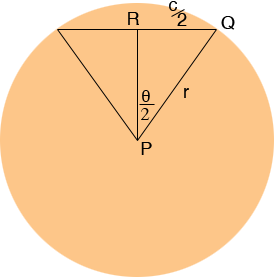SEARCH HOMEMath Central Quandaries & QueriesQuestion from prem: hello sir, i am engineer, i need the formula for calculate the arclength by using only chord length and radius onlyPrem,

The length of an arc of a circle is given by

arclength = r θ

where r is the radius of the circle and θ is the central angle measured in radians.

Suppose the length of the chord is c units. In the diagram below R is the midpoint of the chord.sin(θ/2) = |QR|/|PQ| = c/(2r) and hence θ = 2 sin-1(c/(2r)). Thus

arclength = 2 r sin-1(c/(2r))

HarleyMath Central is supported by the University of Regina and The Pacific Institute for the Mathematical Sciences.